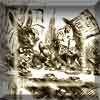#### You may also like### A Problem of Time

Consider a watch face which has identical hands and identical marks for the hours. It is opposite to a mirror. When is the time as read direct and in the mirror exactly the same between 6 and 7?The points P, Q, R and S are the midpoints of the edges of a non-convex quadrilateral.What do you notice about the quadrilateral PQRS and its area?

# Same Length

##### Age 11 to 16Challenge Level

Same Length printable sheet

Below are two diagrams for you to construct.
Measure the lengths - there seem to be some that are equal!
But can you prove it?

Firstly:
Draw a square, and create an equilateral triangle on two adjacent sides of the square.

It looks like the two outside corners of the triangles, along with the opposite corner of the square, form another equilateral triangle... Can you prove it?

What if you constructed the equilateral triangles on adjacent sides of a rectangle, rather than a square?

What if the equilateral triangles are drawn inside the square? Inside the rectangle?

Secondly:

Watch the animation below.

Draw some equilateral triangles of your own. Are the two lines always the same length?
Can you prove it?

With thanks to Don Steward, whose ideas formed the basis of this problem.

The first problem is based on a theorem credited to French mathematician Victor Thebault.